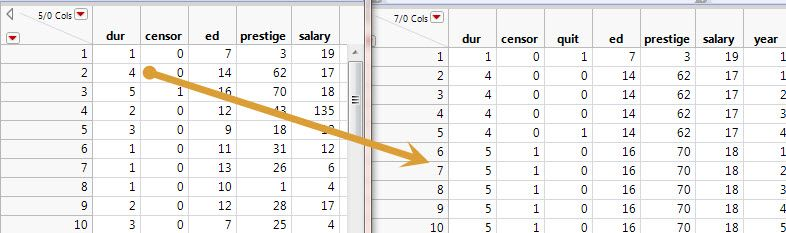Choose Language Hide Translation Bar

## discrete time cox

Hi

Is that possible to perform a Cox Proportional Hazard Regression using discrete time with JMP Pro 12 ?

How to do it with the Fit proportionnal hazard platform ?

In my database, there is one patient per row and i know that the event occured between two visits of the study.

Thanks

Florent

1 ACCEPTED SOLUTION

Accepted Solutions

## Re: discrete time cox

Discrete survival time data is not supported in the Fit Model/Proportional Hazard platform. However, you can run a logistic model after the original survival data is transformed, as shown below.

In this example, the response variable for logisitic regression will be "quit", a 0 and 1 discrete variable, where there are multiple records for those whose survival time (represented by "dur") is more than one year.Please refer to 7.5.2 in https://data.princeton.edu/wws509/notes/c7s6 for details about the data transformation as well as the analogy between the logistic regression and the discrete time proportional hazards model.

3 REPLIES 3

## Re: discrete time cox

Discrete survival time data is not supported in the Fit Model/Proportional Hazard platform. However, you can run a logistic model after the original survival data is transformed, as shown below.

In this example, the response variable for logisitic regression will be "quit", a 0 and 1 discrete variable, where there are multiple records for those whose survival time (represented by "dur") is more than one year.Please refer to 7.5.2 in https://data.princeton.edu/wws509/notes/c7s6 for details about the data transformation as well as the analogy between the logistic regression and the discrete time proportional hazards model.

## Re: discrete time cox

Thank you very much, Jiancao.

In your example, how do you use the "censor" data in the logistic regression model ?

Florent.

## Re: discrete time cox

You’re welcome. You no longer need that since the values of the binary response variable indicate the censoring status.